You are using an outdated browser. Please upgrade your browser to improve your experience.## The resistance calculator is a resistor divider value calculator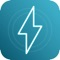# Resistance calculator !

by Murphy Brenna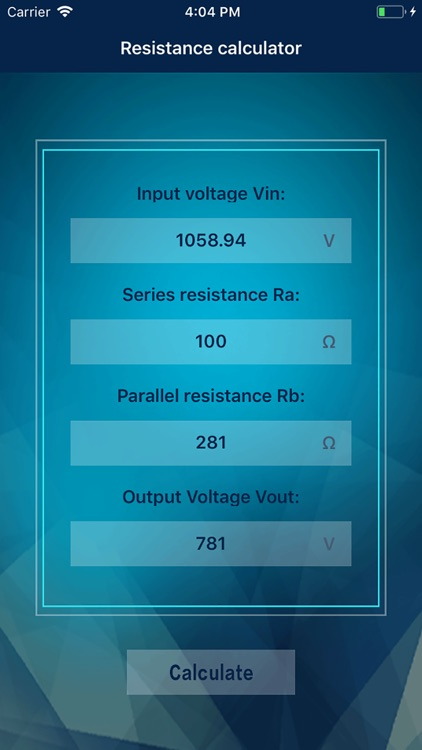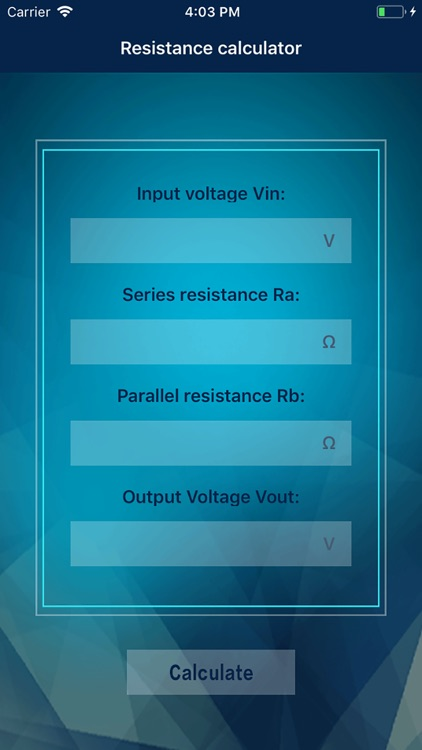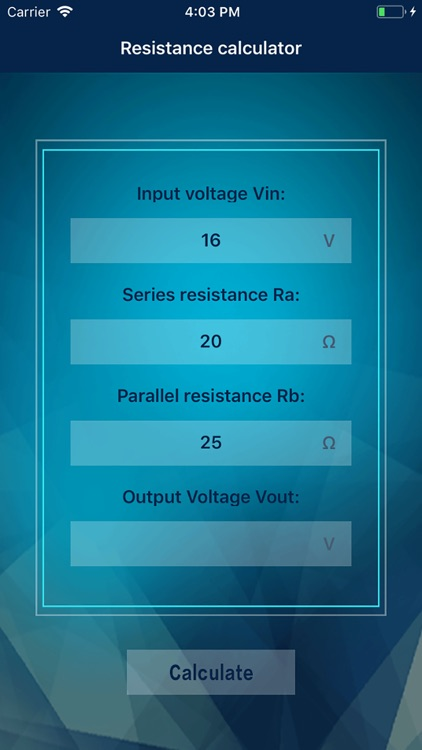### What is it about?

The resistance calculator is a resistor divider value calculator. Electronic engineers often use the voltage divider values of various resistors when debugging circuits, so this provides the fastest calculation method. Simply input a few numbers to get the remaining parameter values.### App Details

Version
1.1
Rating
NA
Size
5Mb
Genre
Utilities
Last updated
September 25, 2018
Release date
September 21, 2018

### App Screenshots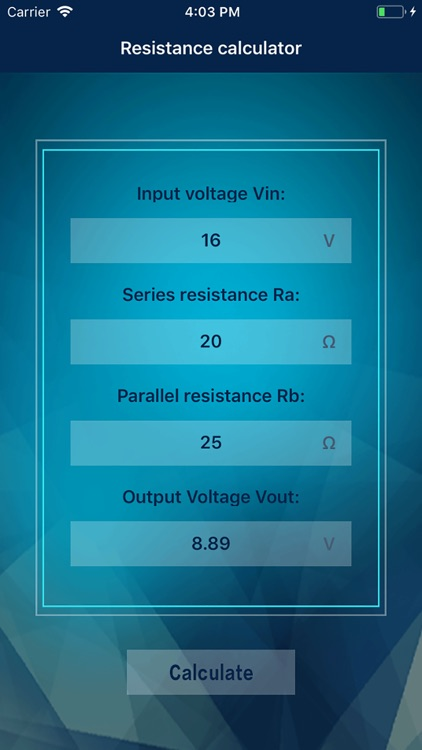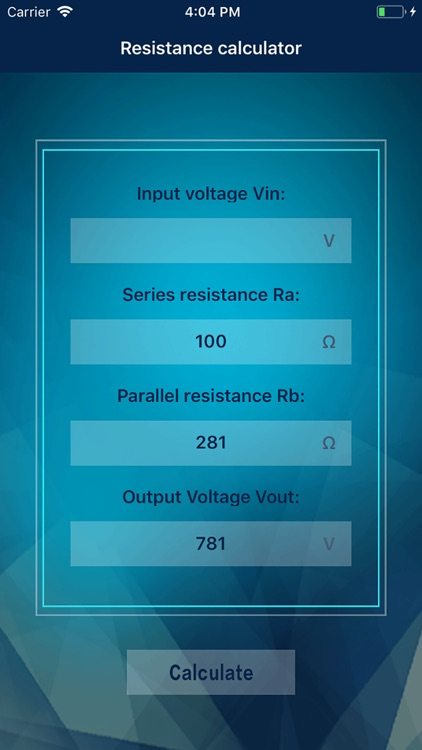### App Store Description

The resistance calculator is a resistor divider value calculator. Electronic engineers often use the voltage divider values of various resistors when debugging circuits, so this provides the fastest calculation method. Simply input a few numbers to get the remaining parameter values.
The so-called partial pressure formula is to calculate how the individual resistors in series divide the total voltage and how much voltage is divided.
The fractional voltage is calculated as: the percentage of the total resistance is the percentage of the divided voltage. The formula is: U = (R / R total) × U source
For example, the 5 ohm and 10 ohm resistors are connected in series in the middle of the 10V circuit, and 5 ohms account for 1/3 of the total resistance of 5+10=15 ohms, so the voltage of the ohm is also 1/3, that is,
10/3 volts.

Disclaimer:
AppAdvice does not own this application and only provides images and links contained in the iTunes Search API, to help our users find the best apps to download. If you are the developer of this app and would like your information removed, please send a request to [email protected] and your information will be removed.